﻿ Optimal Portfolio Selection Using Multi-Objective Fuzzy-Genetic Method

### Optimal Portfolio Selection Using Multi-Objective Fuzzy-Genetic Method

Iman Goroohi Sardou, Ataa Nazari, Esmaeil Ghodsi, Ehsan BagherzadehOPEN ACCESSPEER-REVIEWED

## Optimal Portfolio Selection Using Multi-Objective Fuzzy-Genetic Method

Iman Goroohi Sardou1,, Ataa Nazari1, Esmaeil Ghodsi1, Ehsan Bagherzadeh1

1Faculty of Electrical and Computer Engineering, ShahidBeheshti University, Tehran, Iran

### Abstract

The purpose of investors is to maximize the expected returnin an acceptable level of risk. A genetic algorithm (GA) based on multi-objective fuzzy approach is presented in this paper to solve the multi-objective problem of portfolio selection. The expected return maximization and the risk minimization are the objective functions of the proposed portfolio selection problem. Since GA does not require prespecified information of the problem, it has more flexibility rather than the other nonlinear methods. Furthermore, the GA is able to model the nonlinear manner of the objective functions of the problem. In the proposed fuzzy-genetic method the objective functions are transmitted to a fuzzy domain using a fuzzy membership function and after that the weighted sum method is employed to determine the total objective function. Besides, the Pareto front of the objectives of return and risk are obtained by varying the weighting coefficients and solving the new single-objective problems. To demonstrate the effectiveness of the proposed method, a case study including several active companies is studied.

### At a glance: Figures

12
Prev Next

• Sardou, Iman Goroohi, et al. "Optimal Portfolio Selection Using Multi-Objective Fuzzy-Genetic Method." International Journal of Econometrics and Financial Management 3.2 (2015): 99-103.
• Sardou, I. G. , Nazari, A. , Ghodsi, E. , & Bagherzadeh, E. (2015). Optimal Portfolio Selection Using Multi-Objective Fuzzy-Genetic Method. International Journal of Econometrics and Financial Management, 3(2), 99-103.
• Sardou, Iman Goroohi, Ataa Nazari, Esmaeil Ghodsi, and Ehsan Bagherzadeh. "Optimal Portfolio Selection Using Multi-Objective Fuzzy-Genetic Method." International Journal of Econometrics and Financial Management 3, no. 2 (2015): 99-103.

 Import into BibTeX Import into EndNote Import into RefMan Import into RefWorks

### 1. Introduction

Productive investment is essential for economic growth and development of a country. These are different methods for providing fund to do such investments. Deposits of society members are the main source of providing capital. Therefore, there should be strong facilities for moving the deposits toward production sectors, in order to provide financial requirements.

Although Markowitz model can be solved using mathematical Programing models, its searching space would be too large and inconsistent, when real world limitations, such as large number of capitals, limitation of weighted values of stocks and etc. are added to it. This would practically make mathematical models impossible. That’s why, innovative algorithms such as genetic algorithm, neural networks and ant colony algorithm are of high significance.

Investors’ aim is always earning the maximum expected return in an acceptable level of risk. The concepts of risk and return are two accepted criteria in decision making. All decision makings are based on the interaction between these two factors. The base and reason of diversifying stocks is the fact that general risk of keeping a lot of stocks is lower than the keeping few stocks. As the behavior of stocks in market like many natural phenomena is non-linear and linear models cannot recognize non-linear behavior, but can only well recognize the linear part ofbehavior, non-linear models should be used to recognize stocks behavior. Considering the uncertainties dominating the stock market as well as investors’ tendencies and preferences, it is crucial to find a method for selecting a proper portfolio through which we can overcome the uncertainties and preferences diversity. In some case, investors face ambiguous options that result in incomplete analysis.

 uses value–at–risk method (VAR) to make risk-return balance of capital for optimal selection of portfolio.  proposes a method to evaluate risk and capital return of large loan portfolios. In this method, an equilibrium model is used to reach distribution of loan returns from observed interest rates and certain frequencies.  analyzes different interactions between portfolios limits assuming applying collective restrictions on tracking error volatility (TEV) and value-at-risk(VAR). In  amulti objective particle swarm optimization (MOPSO) has been presented for portfolio selection considering the budget limitation.  proposes a new method to optimize the portfolio selection problem by separating distributions of capital return to two positive and negative half-spaces. This method minimizes a new parameter called partitioned value-at-risk (PVAR) using statistical data of half-space. [6, 7, 8, 9] also present models for optimal selection of portfolio by considering risk and capital return.  uses multi objective model of linear regression to optimal portfolio selection with the objectives of risk minimization and capital return maximization. References [11, 12, 13] refer to portfolio selection optimization for special conditions.

As all investors are risk avoider, they always try to know how much capital return would be obtained for every one-unit of increase in the value of stocks. The return is the profit and benefit due to investment that can be quantitative or qualitative. Return is divided into two section of quantitative (including accountancy profit and capital profit) and quantitative (including company credit, social advantages, power of influencing social and economic trends). Investor act based on return prediction. In creating a portfolio, the relation between risk and return as a collection is important. This point of view is based on statistical and mathematical calculations. Using optimization models and novel portfoliotheory, we can create portfolio which has the least risk opposed to expected return or the maximum return opposed to expected risk. In this paper, genetic algorithm (GA), which reduces the requirement for accurate data, is used to select the best portfolio for short-term investors. Effectiveness of genetic algorithm in optimization problems has made it an appropriate method for investors to select the optimal portfolio. Since genetic algorithm does not need specific information of the problem, it has more flexibility than other methods. Furthermore, genetic algorithm is different from typical searching methods which use stochastic selection to determine their search direction. Although it uses chance and accident to define decision making methods, in the search space of selecting optimal point, it is incidental.

### 2. Problem Formulation

2.1. Markowitz Model

In Markowitz’s portfolio selection method, securities return is considered as random variable. In this model, return and risk are respectively calculated as average and standard deviation of stocks historical returns. The main hypothesis of Markowitz Variance average model is the future situation of securities can be reflected by historical data. It means that return and standard deviation of securities in future is the same as historical data. In fact, in Markowitz model only one aspect of uncertainty, i.e. the incidental feature of stocks, is taken into account. Whereas, in the real world, there are other factors. So only being incidental cannot be guaranteed for return of companies stocks that is always varying. One of the most important innovations of Markowitz was using variance (or standard deviation) of one distribution-probable return distribution-as risk. In other words, he assumed that the higher variance of an investment leads to the more probable difference between the real return and the expected return. By this attitude, Markowitz could convert the tough, complicated and multidimensional problem of portfolio, containing several properties with different features, to a two-dimensional problem analysis; called “average-variance”. Based on Markowitz Formulization, selecting an efficient portfolio, commences with the following three analysis estimations:

A) Expected return for every securities.

B) Variance of expected return for every security.

C) Interaction between securities returns which is measured by covariance between return of every stocks with another.

2.2. Problem Formulization

In the study, one of the most well-known models for portfolio selection. i.e. Markowitz model has been used. Besides, fuzzy-weighting multi-objective method is used to solve the proposed multi-objective portfolio selection problem.

2.2.1. Fuzzy-Weighting Multi-Objective Method

In this method, using weighting method and by defining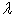and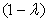as weighing coefficients whichvaries between 0 and 1, the multi-objective problem is converted to a single objective problem, as: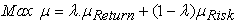(1)

where,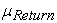is the membership function value of the objective function of return and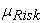is the membership function value of objective function of capital risk, which are calculated using fuzzy method. In this paper, fuzzy method is used to normalize the objective functions. In this paper, the membership functions of eq. (2) and eq. (3) are respectively used for objective functions which should be minimized (risk) and maximized (return):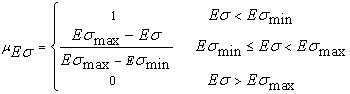(2)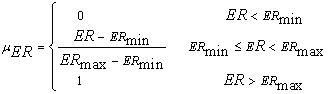(3)

2.2.2 Objective Function
Portfolio return

Portfolio return is calculated as: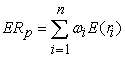(4)

Where n is number of stocks,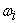is the percentage of each stock and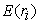is arithmeticmean of return rate related to historical data and is calculated as: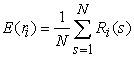(5)

Where N is number of weeks,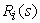is the return of ith stock in sth week that is defined in follows.

Stock return: Return is an award which each stock grants to its owner. It includes income earned from buying and selling of the stocks, debit profit of stocks and precedency and the award of incorporation. It can be computed as: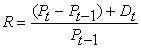(6)

where R is total return of the portfolio, Dt is dividend of the stocks in period t,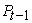is the price of monetary property in the start of period t and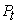is the price of monetary property at the end of period t.

Risk of the portfolio

One of the methods of measuring risk is standard deviation. Standard deviation denotes return deviation from its expected value; i.e. the level of dispersion in expected return. The more dispersion results in the more standard deviation and thereby more risk. While, the less dispersion leads to the less risk. For calculating standard deviation from historical data, eq. (7) can be used.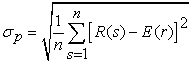(7)

where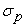is standard deviation.

Finally risk of the portfolio can be calculated as: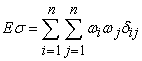(8)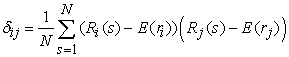(9)

whereis the investment percentage on ith Stocks and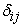is covariance between ith and jth Stocks.

2.2.3. Problem Constraints

The constraints of the portfolio selection problem are defined as: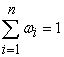(10)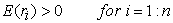(11)

### 3. Solution Methodology

3.1. Genetic Algorithm

Genetic algorithm is inspired by the science of genetic and Darwin evolution hypothesis. It is based on survival of the superiors or natural selection. A prevalent usage of genetic algorithm is as an optimization tool. Genetic algorithm is an effective tool in model recognition, feature selection, perception of depiction and machine learning. In genetic algorithms, genetic evolution of beings is simulated.

Depiction 1: genetic algorithm

In this study, a genetic algorithm is used to optimize the proposed portfolio selection problem. Generally, this algorithm follows the following steps:

1. Determining algorithm parameters such as type of selection, crossover and mutation operators, their rates, the number of iterations, which is considered as the stop criterion of the algorithm.

2. Determining constraints, such as number of stocks

3. Generation of the initial population of genetic algorithm

4. Calculation of the fitness of each member of initial population using the fitness function.

5. Selection of the most superiormembers and their optimal allocation to the optimal population

6. Applying the crossover and mutation operators

7. Considering the satisfaction of the specified constraints

8. Determining the best answer

9. Considering if the algorithm stop criterion is satisfied or not?

10. In the case of satisfying the stop criterion of the algorithm, it is stopped; otherwise it repeats from step 4.

The flowchart of genetic algorithm is shown in Figure 1.

### 4. Numerical Results

In this paper, the efficiency of the proposed method is demonstrated using a practical case study containing the stocks of seven large commercial companies. It includes weekly prices in the period from September 1, 2012 to January 1, 2013. Information is derived from Yahoo server. Names of the companies and average return of the stocks are shown in Table 1.

#### Table 1. Information of the case study

Figure 2 illustrates the weekly return curve for the companies. As just companies with positive return average are considered for the portfolio selection, companies of ATI, IBM, MSFT and yahoo are investigated as candidates for portfolio selection in the case study. The genetic algorithm used in this case study is single stage genetic algorithm. The used selection technique is roulette wheel mechanism. In this algorithm uniform mutation operator with rate of 0.05 and medial crossover operator with rate of 0.9 is employed. The size of population and the iteration number is considered as 100 and 300, respectively.

The simulation is done by version 7.10 of MATLAB software. By solving the problem for (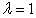), minimum and maximum values of return are specified as 0.00344 and 0.00864, respectively. Besides, solving the problem for (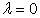) results in minimum and maximum risk of 0.018388 and 0.06546, respectively. In the following, the result of solving the problem for two cases are presented:

Case 1: determining the most preferred solution, considering equal importance for return and risk (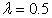).

Case 2: Determining Pareto optimal solutions set and Pareto front (by varying).

4.1. Case 1

Considering the same importance for both objective functions of return and risk (), the results of the optimal portfolio are shown in Table 2. Figure 3 shows the convergence manner of genetic algorithm for Case.

4.2. Case 2

In this case, the value of, which denotes the importance coefficient of return objective function, is changed from minimum of 0 to maximum of 1. By selecting 100 different values with equal distances forand solving the problem of optimal portfolio selection for eachvalue, optimal Pareto front is achieved for return and risk objective functions, as Figure 4. Based on the decision maker’s point of view, each of the points of the Pareto front curve can be considered as the final solution of the portfolio selection problem.

### 5. Conclusion

In this paper, genetic algorithm is used to determine the optimal portfolio for short-term investors according to their tendency toward having the most return and the least risk in investment. Generally following conclusions can be made from this study:

1. Based on genetic algorithm, there is an inverse relationship between diversification in stocks and portfolio risk.

2. Based on genetic algorithm, effectiveness of diversification in portfolio increases with increasing the number of portfolio stocks for short-term investors.

3. Genetic algorithm is efficient in optimal portfolio selection.

4. There is difference between return of incidental portfolio and that of genetic algorithm portfolio.

### References

  X. Sun, J. Li, L. Tang, and D. Wu. Identifying the risk-return tradeoff and exploring the dynamic risk exposure of country portfolio of the FSU's oil economies, Economic Modelling, 29. (2012). pp. 2494-2503.In article CrossRef  J. Mencía. Assessing the risk-return trade-off in loan portfolios, Journal of Banking & Finance, 36. (2012). pp. 1665-1677.In article CrossRef  G. Palomba, Luca Riccetti. Portfolio frontiers with restrictions to tracking error volatility and value at risk, Journal of Banking & Finance, 36. (2012). pp. 2604-2615.In article CrossRef  S. Kumar Mishra, G. Panda,R. Majhi.A comparative performance assessment of a set of multiobjective algorithms for constrained portfolio assets selection, Swarm and Evolutionary Computation, 16.(2014). pp. 38-51In article CrossRef  Joel WeiqiangGoh, Kian Guan Lim, Melvyn Sim, Weina Zhang. Portfolio value-at-risk optimization for asymmetrically distributed asset returns, European Journal of Operational Research, 221. (2012). pp. 397-406.In article CrossRef  R. J. Bianchi, G. Bornholt, M. E. Drew, M. F. Howard. Long-term U.S. infrastructure returns and portfolio selection, Journal of Banking & Finance, 42. (2014). pp. 314-325.In article CrossRef  Wei. Zhang, Q. Mei, Q. Lu, W. Xiao. Evaluating methods of investment project and optimizing models of portfolio selection in fuzzy uncertainty, Computers & Industrial Engineering, 61. (2011). pp. 721-728.In article CrossRef  C. Aranha, C. R.B. Azevedo, H. Iba. Money in trees: How memes, trees, and isolation can optimize financial portfolios, Information Sciences, 182. (2012). pp. 184-198.In article CrossRef  Min Zhu, Return distribution predictability and its implications for portfolio selection, International Review of Economics & Finance, 27 (2013). pp. 209-223.In article CrossRef  F. Makhatabrafiei, M.A. Fatahzadeh, Linear regression and multiobjective method to solve the portfolio selection problem, 9th international conference of industrial engineering, Tehran, Iran, 2013.In article  X. Li, Z. Qin, Interval portfolio selection models within the framework of uncertainty theory, Economic modeling, 41 (2014), pp. 338-344.In article CrossRef  H. Yao, Z. Li, Sh. Chen, Continuous-time mean-variance portfolio selection with only risky assets, Economic modeling, 36 (2014), pp. 244-251.In article CrossRef  F. He, R. Qu, A two-stage stochastic mixed-integer program modeling and hybrid solution approach to portfolio selection problems, Information Sciences, (2014), In press.In article CrossRef